LaTeX完成Scientific Papers的一些说明

• A+

简介

更多资料

LaTeX的更多详细说明 : LaTeX指南信息

表格的绘制

• hline表示横线
• toprule: 表示三线表的表头的横线(这个与后面两个三线表的都需要使用booktabs
• midrule: 表示三线表中间的横线
• bottomrule: 表示三线表最后的横线

1. \documentclass{article}
2. \usepackage{booktabs}
3. \begin{document}
4. % --
5. \begin{table}
6.     \centering
7.     \begin{tabular}{lcccc}
8.         \toprule
9.         & \multicolumn{4}{c}{Data} \\ \cmidrule(lr){2-5}
10.         DoS Types & Correct &  Wrong & Total & Accuracy \\
11.         \midrule
12.         back & 0 & 359 & 359 & 0.00\% \\
13.         land & 7 & 0 & 7 & 100.00\% \\
14.         neptune & 4616 & 41 & 4657 & 99.12\% \\
15.         pod & 36 & 5 & 41 & 87.80\% \\
16.         smurf & 652 & 13 & 665 & 98.05\% \\
17.         teardrop & 12 & 0 & 12 & 100\% \\
18.         mailbomb & 0 & 293 & 293 & 0.00\% \\
19.         apache2 & 72 & 665 & 737 & 9.77\% \\
20.         processtable & 135 & 550 & 685 & 19.71\% \\
21.         udpstorm & 0 & 2 & 2 & 0.00\% \\
22.         \bottomrule
23.     \end{tabular}
24.     \caption{The Model1 for DoS Attack}
25.     \label{tab-label}
26. \end{table}
27. \end{document}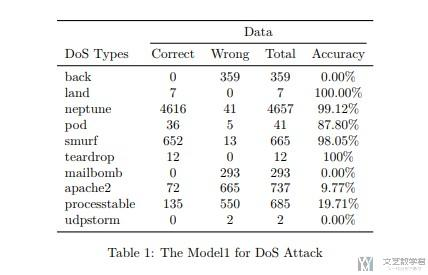表格的自动换行

1. \begin{table}[htbp!]
2.     % 我提取的特征与其他人提取的特征
3.     \centering
4.     \begin{tabular}{|p{2cm}|p{5cm}p{2cm}p{2cm}|}
5.         \toprule
6.         Attack Types & Important Features in cite &  Numbers of Important Features in cite & The Same Important Feature \\
7.         \midrule
8.         DoS & 3, 4, 5, 6, 8, 23, 29, 36, 38, 39, 40 & 11 & 9 \\
9.         Probe & 2, 3, 4, 5, 6, 12, 29, 32, 33, 34, 35, 36, 37, 40 & 14 & 11 \\
10.         R2L & 1, 2, 3, 5, 6, 10, 12, 14, 16, 24, 32, 33, 35, 36, 37, 38, 39, 41 & 18 & 9 \\
11.         U2R & 3, 5, 6, 10, 14, 17, 32, 33 & 8 & 6 \\
12.         \bottomrule
13.     \end{tabular}
14.     \caption{Performances of The Model}
15.     \label{tab5-ModelExplain-1}
16. \end{table}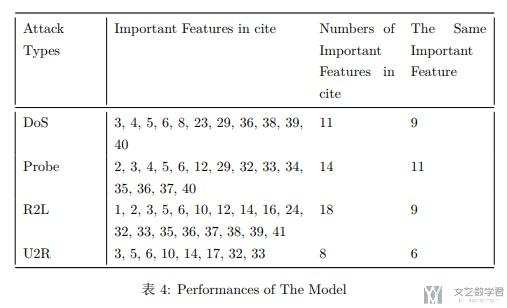长表格自动换页

1. \documentclass{article}
2. \usepackage{longtable}
3. \begin{document}
4. \begin{longtable}{|c|c|r|r|r|r|}
5. \caption{caption}
6. \label{table:label} \\ % add \\ command to tell LaTeX to start a new line
7. % Appear table header at the first page as well
8. \hline
9. line1 & line2 & $t_1$ & $t_{12}$ & $t_2$ & $r$(\%)\\
10. \hline
12. % Appear the table header at the top of every page
13. \hline
14. line1 & line2 & $t_1$ & $t_{12}$ & $t_2$ & $r$(\%)\\
15. \hline
17. % Appear \hline at the bottom of every page
18. \hline
19. \endfoot
20. % data begins here
21. 10 & 2 & 0:22:00 & 9:46:00 & 2:00:00 & 80.49 \\
22. 204 & 205 & 2:01:00 & 2:57:00 & 1:11:00 & 47.97 \\
23. % 这里复制粘贴更多的数据, 使其可以超出一页的范围
24. \hline
25. \end{longtable}
26. \end{document}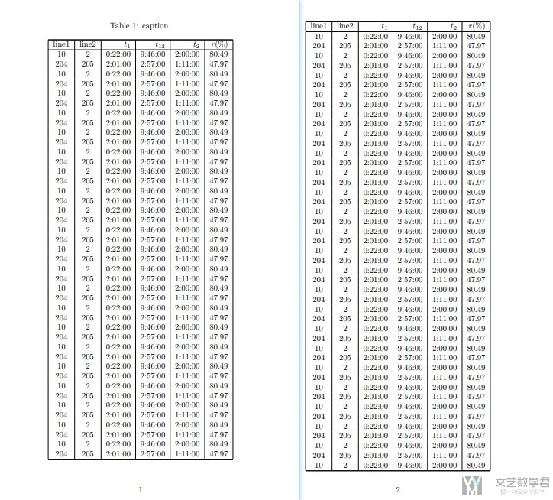表格种内容的对齐

1. \usepackage{array}

1. \begin{table}
2.      \begin{center}
3.      \label{pntable}
4.      \begin{tabular}{|p{2cm}<{\raggedright}|>{\centering}p{6.5cm}|p{3cm}<{\raggedleft}|}
5.      \hline
6.      \textbf{Integer} & \textbf{Partitions} & \textbf{Number of partitions} \\ \hline
7.      1 & 1 & 1 \\ \hline
8.      2 & 2, 1+1 & 2 \\ \hline
9.      3 & 3, 2+1, 1+1+1 & 3 \\ \hline
10.      \end{tabular}
11.      \end{center}
12.      \caption{All the partitions of $n \in \{1,\ldots,3\}$}
13. \end{table}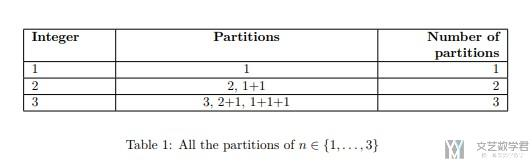引用subfigure

1. \documentclass{article}
2. \usepackage{subfigure}
3. \usepackage{graphicx}
4. \usepackage{caption,setspace}
5. \begin{document}
6. \begin{figure*}[htbp!]
7.     \centering
8.     \subfigure[Explanation for DoS]{
9.         \includegraphics[width=4.16in]{DoSAttack.png} % 二分类模型的解释, DoS攻击的数据
10.         \label{fig5-Binary-1:1}
11.     }
13.     \subfigure[Explanation for Normal]{
14.         \includegraphics[width=4.16in]{Normal.png} % 二分类模型的解释, Normal数据
15.         \label{fig5-Binary-1:2}
16.     }
17.     \caption{Specific Data Explanation}
18.     \label{fig5-Binary-1}
19. \end{figure*}
20. Fig.~\ref{fig5-Binary-1}
21. Fig.~\ref{fig5-Binary-1:1}
22. Fig.~\ref{fig5-Binary-1:2}
23. \end{document}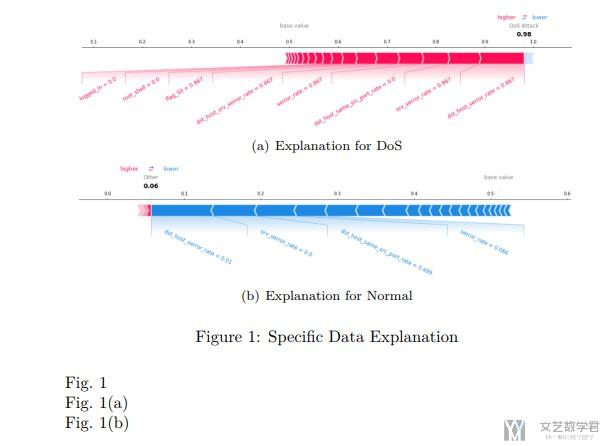公式的书写

1. \documentclass{article}
2. \usepackage{amsmath}
3. \begin{equation}
4.     F \text { -Score }=\frac{2 * \text { Recall } * \text { Precision }}{\text { Recall }+\text { Precision }}
5. \end{equation}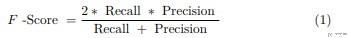一些常用的数学公式

1. \frac{N}{M}1. \sum_{i \in (1,100)}^{100}1. \overline{\phi_{i}(f, x)} \qquad \underline{\phi_{i}(f, x)}m行n列图片插入(子图插入)

1. \usepackage{subfigure}
2. \begin{figure}[htbp!]
3.     \centering
4.     \subfigure[dst\_host\_serror\_rate Distribution]{
6.     }
7.     \subfigure[srv\_serror\_rate Distribution]{
9.     }
11.     \subfigure[wrong\_fragment Distribution]{
13.     }
14.     \subfigure[wrong\_fragment Distribution]{
16.     }
17.     \caption{The disturbition of Data}
18.     \label{fig5-Distribution-1}
19. \end{figure}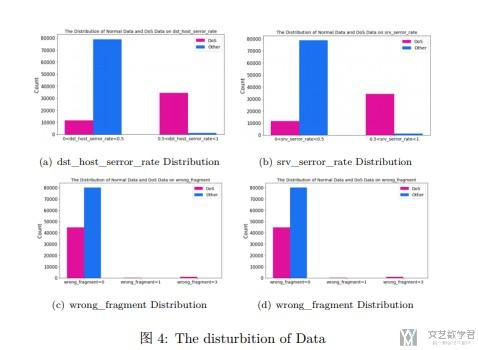交叉引用

引用章节

1. \documentclass{article}
2. \usepackage[utf8]{inputenc}
3. \usepackage{ctex} % 对于中文的显示
4. \usepackage{booktabs}
5. \begin{document}
6. % 引用章节
7. \section{123}
8. \subsection{abc} \label{abc}
9. \subsection{def}
10. 这里引用subsection\{abc\}的内容, \ref{abc}.
11. \end{document}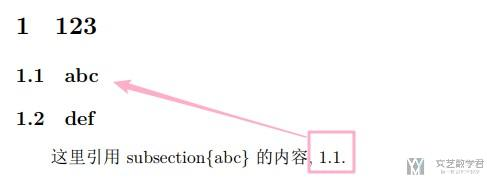论文的引用

1. % 参考文献
2. \bibliographystyle{unsrt}
3. \bibliography{ref} % 存文字的名字

• plain，按字母的顺序排列，比较次序为作者、年度和标题
• unsrt，样式同plain，只是按照引用的先后排序
• alpha，用作者名首字母+年份后两位作标号，以字母顺序排序
• abbrv，类似plain，将月份全拼改为缩写，更显紧凑：
• ieeetr，国际电气电子工程师协会期刊样式：
• acm，美国计算机学会期刊样式：
• siam，美国工业和应用数学学会期刊样式：
• apalike，美国心理学学会期刊样式：

引用多处文献

1. \usepackage{cite}
2. \cite{bibtex1，bibtex2，bibtex3}

引用公式

1. \begin{equation} \label{part3_eq2}
2.     x^{\prime}
3. \end{equation}
4. % 上面定义了Label, 下面就可以引用
5. This is \ref{part3_eq2}.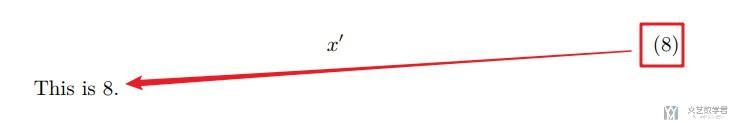LaTeX手动换行

1. long\allowbreak word
2. long\linebreak word

1. \renewcommand\_{\textunderscore\allowbreak} % 使得_可以换行

• 微信公众号
• 关注微信公众号
•• QQ群
• 我们的QQ群号
•